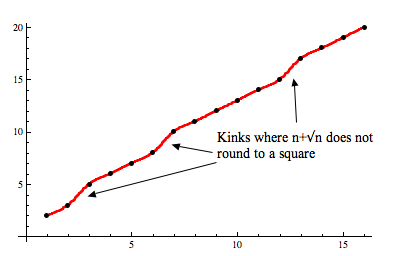# Why, when we round n+sqrt(n), do we never get a square?

Posted by: Gary Ernest Davis on: November 28, 2010

Jim Tanton (@jamestanton) Tweeted recently that for a positive integer$n, n+\sqrt{n}$, rounded to the nearest integer, is never the square of an integer.This certainly seems to be borne out by a few calculations (carried out in Excel, using the built-in “round’ function):

 n n+sqrt(n) round(n+sqrt(n)) 1 2 2 2 3.414213562 3 3 4.732050808 5 4 6 6 5 7.236067977 7 6 8.449489743 8 7 9.645751311 10 8 10.82842712 11 9 12 12 10 13.16227766 13 11 14.31662479 14 12 15.46410162 15 13 16.60555128 17 14 17.74165739 18 15 18.87298335 19 16 20 20 17 21.12310563 21 18 22.24264069 22 19 23.35889894 23 20 24.47213595 24 21 25.58257569 26 22 26.69041576 27 23 27.79583152 28 24 28.89897949 29 25 30 30 26 31.09901951 31 27 32.19615242 32 28 33.29150262 33 29 34.38516481 34 30 35.47722558 35 31 36.56776436 37 32 37.65685425 38 33 38.74456265 39

We see that the square numbers 4, 9, 16, 25 and 36 are indeed missing from this table.

Why should this be so?

I am going to use an argument due to David Ratcliffe (@daveinstpaul)Â  that splits into two different cases and uses an argument by contradiction, which is part of a series of techniques that mathematicians use that go by the general name of “counterfactuals”.

Terry Tao has written extensively about these sort of arguments, The general idea is that we set up a world that we believe cannot exist – sort of like in science fiction, but math fiction. Then we carefully examine this world for consequences and see if something truly unacceptable occurs. If it does, we conclude that no such world can exist.

The world we set up is one in which, contrary to our empirical observations, there is an instance of a positive integer$n$ for which rounding$n+\sqrt{n}$ to the nearest integer gives us a square number, which we will name$k^2$.

What does rounding$n+\sqrt{n}$ to get$k^2$ mean, in a way that allows us to think about consequences?

It means that$k^2-\frac{1}{2}< n+\sqrt{n} < k^2+\frac{1}{2}$.

How can we use this imaginary scenario to draw conclusions, hopefully very strange ones?

Let’s think about the following two mutually exclusive cases, at least one of which must hold good:

1.$n\leq k^2-k$.

In this case imagine, counterfactually, that$\sqrt{n}\geq k-\frac{1}{2}$.

Then$n \geq (k-\frac{1}{2})^2=k^2-k+\frac{1}{4}> k^2-k$.

This contradictory state of affairs means that, in this case, we have$\sqrt{n}.

Then$n+\sqrt{n}< (k^2-k)+(k-\frac{1}{2})=k^2-\frac{1}{2}$

2.$n > k^2-k$.

In this case, because$n$ is an integer, we have$n\geq k^2-k+1$.

In this case, imagine, again counterfactually, that$\sqrt{n}\leq k-\frac{1}{2}$.

Then$n \leq (k-\frac{1}{2})^2=k^2-k+\frac{1}{4}$, contrary to$n \geq k^2-k+1$.

Again, this contradictory state of affairs means that, in this case, we have$\sqrt{n}>k-\frac{1}{2}$.

Then$n+\sqrt{n}> (k^2-k+1)+(k-\frac{1}{2})=k^2+\frac{1}{2}$.

In either case,$n+\sqrt{n}$ does not lie between$k^2-\frac{1}{2}\textrm{ and } k^2+\frac{1}{2}$ as we assumed counterfactually.

This contradictory state of affairs means that, contrary to what we asserted in our counterfactual world, we can never round$n+\sqrt{n}$ to obtain the square of an integer.

### 2 Responses to "Why, when we round n+sqrt(n), do we never get a square?"Nice!

I did some work on this in R on the difference between n + n**.5 and k^2. It follows an interesting pattern, peaking and valleying. The relative minima get arbitrarily close to .5.

CODE STARTS
a <- 100000
n <- 1:a
nrn <- n + n^.5
nsq <- n^2

diff <- vector('numeric', 100)
for (i in 1:a){
diff[i] <- min(abs(nrn[i] – nsq))
}

CODE ENDS

plot(n, diff)

plot(n[diff<.6], diff[diff < .6])

plot(n[position], diff[position])

The first two are better with a smaller range of N.

By n = 100,000 the smallest minimum is pretty close to .5 – it is 0.500396[…] Republic of Mathematics Blog has a nice indirect proof of a problem that James Tanton posted on twitter, that when we round n+sqrt(n), for integer n, we […]